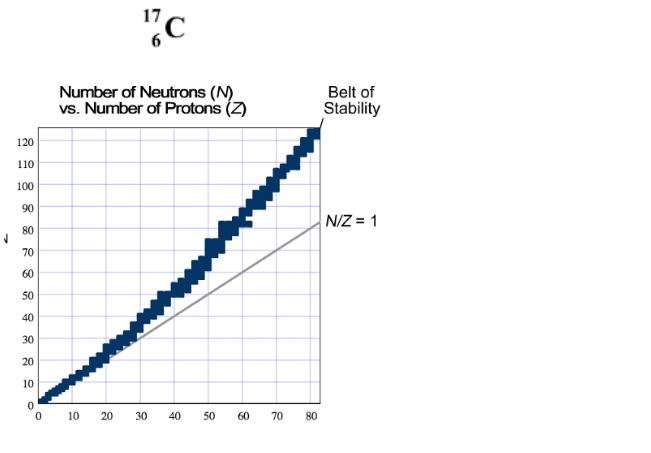Problem: Which type of nuclear decay is the following radioactive isotope likely to undergo?a. alpha decayb. beta decayc. positron emission or electron capture

FREE Expert Solution
100% (465 ratings)
Problem Details

Which type of nuclear decay is the following radioactive isotope likely to undergo?

a. alpha decay

b. beta decay

c. positron emission or electron captureWhat scientific concept do you need to know in order to solve this problem?

Our tutors have indicated that to solve this problem you will need to apply the Band of Stability concept. You can view video lessons to learn Band of Stability Or if you need more Band of Stability practice, you can also practice Band of Stability practice problems .

What is the difficulty of this problem?

Our tutors rated the difficulty of Which type of nuclear decay is the following radioactive iso... as medium difficulty.

How long does this problem take to solve?

Our expert Chemistry tutor, Dasha took 4 minutes to solve this problem. You can follow their steps in the video explanation above.

What professor is this problem relevant for?

Based on our data, we think this problem is relevant for Professor Espinoza's class at UIC.